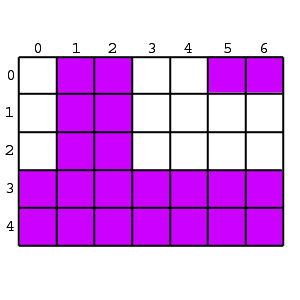### Problem Statement

Given a rectangular grid where all the cells are initially white, you perform the following procedure K times:

• Select a cell from the grid at random, and call it A
• Select a cell from the grid at random, and call it B
• Color all the cells in the rectangle bounded by A and B
Each cell is selected at random, with uniform distribution, and each selection is independent from the other selections. It's possible that A and B correspond to the same cell.

For example, the image below shows a 5x7 grid where the selected pairs could have been (row, column):

• (0,1); (3,2)
• (3,6); (4,0)
• (0,6); (0,5)
Resulting in a grid with 22 colored cells and 13 white cells.You are given ints rows and cols, the dimensions of the grid. Return the expected number of colored cells after K steps.

### Definition

 Class: GridColoring Method: area Parameters: int, int, int Returns: double Method signature: double area(int K, int rows, int cols) (be sure your method is public)

### Notes

-The returned value must be accurate to within a relative or absolute value of 1E-9.

### Constraints

-K will be between 0 and 100, inclusive.
-rows will be between 1 and 1000, inclusive.
-cols will be between 1 and 1000, inclusive.

### Examples

0)

 `1` `2` `1`
`Returns: 1.5`
 The grid has two cells. The probability that both of them will get colored is 0.5, and the probability that only one of them will get colored is 0.5. So the expected value is 0.5 * 2 + 0.5 * 1 = 1.5.
1)

 `2` `2` `1`
`Returns: 1.875`
 With the same grid as in the previous example, but two selections, the expected value rises to 1.875.
2)

 `1` `2` `2`
`Returns: 2.25`
3)

 `3` `5` `7`
`Returns: 19.11917924647044`

#### Problem url:

http://www.topcoder.com/stat?c=problem_statement&pm=8012

#### Problem stats url:

http://www.topcoder.com/tc?module=ProblemDetail&rd=12181&pm=8012

slex

#### Testers:

PabloGilberto , Olexiy , ivan_metelsky , ged

Math